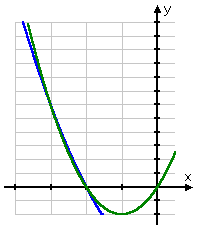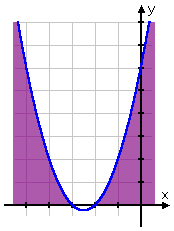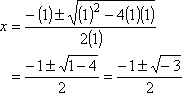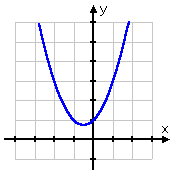Search

Solving Quadratic Inequalities: Examples (page 2 of 3)

• Solve 2x2 + 4x >   x2  x  6.

The two associated two-variable equations in this case are y = 2x2 + 4x and y = x2  x  6.

 This inequality is asking when the parabola for y = 2x2 + 4x (in green) is higher than the parabola for y = x2  x  6 (in blue):As you can see, it is hard to tell where the green line (y = 2x2 + 4x) is above the blue line
(
y = x2  x  6). So, instead of trying to solve this inequality, I will instead work with the following related inequality:

2x2 + 4x > x2  x  6
2x2 + 4x  x2 + x + 6 > 0

x2 + 5x + 6 > 0

This last inequality is simpler to deal with because now all I have to do is find the zeroes of y = x2 + 5x + 6 (which is easy) and then pick the correct intervals based on just the one parabola (which is also easy). That is, it is simpler to compare one parabola with the x-axis than to compare two parabolas with each other. But since the one parabola (y = x2 + 5x + 6) came from combining the two original parabolas ("paraboli"?), the solution to the simpler one-parabola inequality will be the same as the solution to the original two-parabola inequality. Since the solutions will be the same, I'll work with the simpler case.

I have simplified "2x2 + 4x > x2  x  6" to get "x2 + 5x + 6 > 0". The associated two-variable equation is y = x2 + 5x + 6. First, I'll find the zeroes (that is, the x-intercepts):

Advertisement

x2 + 5x + 6 = 0
(x + 2)(x + 3) = 0

x = 2
or  x = 3

These two intercepts split the number-line into three intervals, namely x < 3, 3 < x < 2, and x > 2. On which of these three intervals is y = x2 + 5x + 6 above the x-axis? Since y = x2 + 5x + 6 graphs as a right-side-up parabola, the quadratic is above the axis on the ends:Then the solution is:

x < 3  or  x > 2

Why was this solution "or equal to", rather than just "greater than" or "less than"? Because the original inequality was "or equal to", so the boundary points, being the zeroes or x-intercepts, are included in the solution.   Copyright © Elizabeth Stapel 2002-2011 All Rights Reserved

• Solve x2 + x + 1 > 0.

First, I'll find the zeroes:Hmm... Since there is a negative inside the square root, there must not be any x-intercepts. That is, this quadratic must be either always above the x-axis or else always below, because it can never cross or touch the axis.

 Since y = x2 + x + 1 is a "positive" quadratic, the parabola is right-side-up, so I know it goes up forever. For the parabola not to cross the x-axis, it must be that the parabola is always above the axis, as you can see here:So when is y = x2 + x + 1 greater than zero (above the axis)? Always! Then the solution is:

all x

The above solution could also be stated as "all real numbers" or written as the interval "from negative infinity to positive infinity".

• Solve x2 + x + 1 < 0.

This looks just like the previous problem, except that now I'm looking for where the parabola is below the axis. I already know that there are no x-intercepts. Also, because this is a right-side-up parabola, I know that the graph is always above the axis. So where is y = x2 + x + 1 less than zero? Nowhere! Then the solution is:

no x

The above solution could also be stated as "no solution" or as "the empty set", represented by the character "Ø".

Whenever you have a quadratic inequality where the associated quadratic equation does not have real solutions (that is, where the associated parabola does not cross the x-axis), the solution to the inequality will either be "all x" or "no x", depending upon whether the parabola is on the side of the axis that you need.

<< Previous  Top  |  1 | 2 | 3  |  Return to Index  Next >>

 Cite this article as: Stapel, Elizabeth. "Solving Quadratic Inequalities: Examples." Purplemath. Available from     https://www.purplemath.com/modules/ineqquad2.htm. Accessed [Date] [Month] 2016

Study Skills Survey

Tutoring from Purplemath
Find a local math tutor

 Copyright © 2021  Elizabeth Stapel   |   About   |   Terms of Use   |   Linking   |   Site Licensing Contact Us